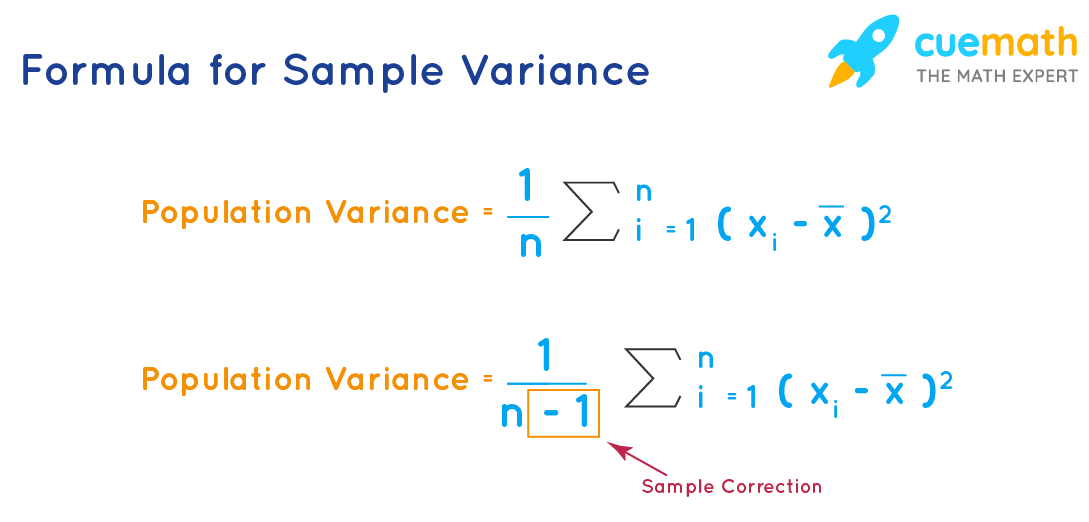# Sample Variance Formula

Before going to learn the sample variance formula, let us recall what is sample variance. In a practical situation, when the population size N is large it becomes difficult to obtain value xi for every observation in the population and hence it becomes difficult to calculate the variance for the population. In such cases, we can estimate the variance by calculating it on a sample of size n taken from the population of size N. This estimated variance is called the sample variance (S2). Let us explore the sample variance formula for a given sample below.

## What Is the Sample Variance Formula?

The sample variance formula involves the sample size and the mean. Given a sample of data (observations) for the random variable x, its sample variance is given by

$$S^2 = \dfrac{1}{n-1} \sum^{n}_{i=1}(x_i - \bar{x})^2$$

where

• S2 is the sample variance
• n is the sample size
• xi is the ith value of the random variable x and is the sample mean.Let us see the applications of the sample variance formula in the following section.

## Solved Examples Using the Sample Variance Formula

### Example 1: There are 45 students in a class. 5 students were randomly selected from this class and their heights (in cm) were recorded as follows:

 131 148 139 142 152

### Calculate the sample mean and the sample variance of their heights (in cm).

Solution:

Sample size (n) = 5

Sample Mean = $$\dfrac{131 + 148 + 139 + 142 + 152}{5}$$ = $$\dfrac{712}{5}$$ = 142.4 cm

Using the sample variance formula,

Sample Variance = $$\dfrac{1}{n-1} \sum^{n}_{i=1}(x_i - \bar{x})^2$$ = $$\dfrac{1}{5-1} \sum^{5}_{i=1}(x_i - 142.4)^2$$

= $$\dfrac{(131 - 142.4)^2 + (148 - 142.4)^2 + (139 - 142.4)^2 + (142 - 142.4)^2 + (152 - 142.4)^2}{4}$$ = 66.3 cm2

Answer: Sample Mean = 142.4 cm, Sample Variance = 66.3 cm2

### Example 2: There are 8 small-sized trees in a garden. Given below are the heights (in cm) of these trees. (Take approximations wherever necessary)

 812 836 982 769 920 1021 942 720

### b. Three trees were randomly chosen from this population and their heights are listed as follows. Find the sample standard deviation of their heights. (Use √2594 = 51)

 1021 982 920

Solution:

a. Population size (N) = 8

Let Xi be the height of the ith tree.

Population Mean () = $$\dfrac{812+836+982+769+920+1021+942+720}{8}$$ = 875.26 cm

Population Variance = $$\dfrac{\sum^{N}_{i=1} (X_i - \bar{X})}{N}$$ = $$\dfrac{\sum^{8}_{i=1} (X_i - 875.26 )}{8}$$ =

$$\frac{(812 - 875.26 )^2 + (836 - 875.26 )^2 + (982 - 875.26 )^2 + (769 - 875.26 )^2 + (920 - 875.26 )^2 + (1021 - 875.26 )^2 + (942- 875.26 )^2 + (720 - 875.26 )^2}{8}$$ = 9999.8 cm2 ≈ 10000 cm2

Population Standard Deviation = $$\sqrt{10000}$$ = 100 cm

b. Sample size (n) = 3

Let xi be the height of the ith tree.

Sample Mean () = $$\dfrac{1021+982+920}{3}$$ = 974.3 cm

Using the sample variance formula,

Sample Variance = $$\dfrac{\sum^{n}_{i=1} (x_i - \bar{x})}{n-1}$$ = $$\dfrac{\sum^{3}_{i=1} (x_i - 974.3)}{3-1}$$ = $$\dfrac{(1021 - 974.3)^2 + (982 - 974.3)^2 + (920 - 974.3)^2 + }{2}$$ = 2594.3cm2

Sample Standard Deviation = $$\sqrt{2594.3}$$ = 51 cm

Answer: (a) The population standard deviation of the height of the trees is approximately 100 cm. (b) The sample standard deviation of the height of the trees is approximately 51 cm.